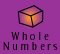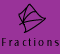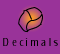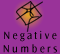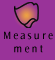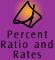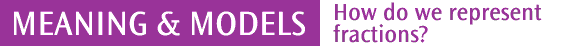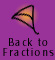| What do we mean by fractions? | Models of fractions |
| The whole and the unit | Quick quiz |

What do we mean by fractions?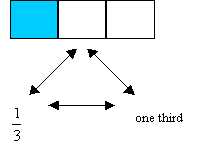A fraction is a number that can be written as a/b ( an indicated quotient), where a is an integer and b is an integer other than zero. Some examples of fractions are: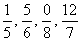Numbers such as 11, -13 and 0 are also fractions. They can be written in this way: 11/1 , -13/1, 0/1 or 0/4. Not all numbers are fractions: the square root of 2 is not a fraction and neither is pi (although it is often approximated by 22/7).

The word fraction comes from a Latin word meaning to break, suggesting that initially a fraction may have been viewed as part of a broken whole.

This early view of the meaning of a fraction is evident when fractions are thought of as expressing a part to whole relationship, where the whole is divided into parts of equal value. There are other meanings for fractions but this way of making sense of them is the one usually used in primary school mathematics.

When representing fractions based on this meaning with materials or diagrams the object for the whole may be one single object or area of a figure. However, the whole can also be made up a collection of objects or areas of several figures together. The models of fractions below illustrate some of these examples.

In either case, the number represented by some part of the whole is always done in reference to the unit.

The fraction 2/5, for example, indicates that the reference unit (the "whole") has been divided into 5 equal parts and 2 of these parts make up this fraction.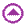Models of fractions

We can use different models to represent fractions.

A whole and therefore a unit can be,

• a region or area of an object

"Can I have a quarter of your sandwich?"• a length of something which is divided into equal parts of the same length

"I need to cut that piece of wood into 3 equal lengths, that is, into thirds"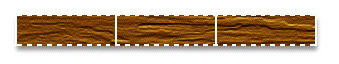• a set of objects divided into equal parts, that is subsets, which each have the same number of objects

"I have 18 tennis balls to be shared equally among 6 children. Each will get one sixth of the tennis balls."The whole and the unit

When the whole equals one, the unit also equals one.

Example 1: A fraction as a part to whole relationship when the whole is one unit.

Show two thirds, 2/3, of a rectangle?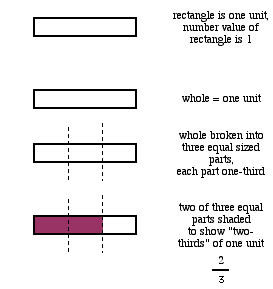When the whole is more than one, we think of the whole as being made up of more than one unit.

Example 2: A fraction as a part to whole relationship when the whole is more than one unit.

Describe two thirds (2/3) of 2 rectangles?

We want to find 2/3 of 2 rectangles, so in this case "the whole" is 2 rectangles and we will call each rectangle "a unit".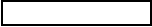rectangle is 1 unitwhole = 2 rectangles or we could say 2 unitsWe can easily find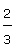of each unit. We divide each unit into 3 equal sized parts, that is into thirds and then shade 2 parts.of 1 unit is.

Soof 2 units can be shown by+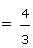By rearranging we can see that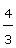is equivalent to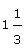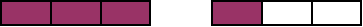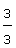+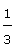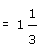Answer: 2/3 of 2 rectangles is 1 1/3 rectangles.

The example above demonstrates the importance of distinguishing the whole and the units that are used to describe the whole. Any quantity can be interpreted as "the whole" (as in this case, two rectangles).Quick quiz

1. Write a fraction for 5 out of 9 equal parts.
2.

On a piece of paper, indicate how you would shadeof the following shape.

3.

Shadeof the following shape4.

Tess was asked to shade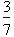of the following shape.

She divided the shape into 7 parts and shaded 3 of them as follows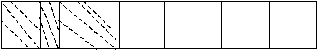Is she correct? Explain why.

5.What fraction of the marbles above is blue?

6.

Shadeof the following shape.7.

How many apples would be left if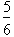of them were eaten?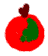8.

How many of the following smiley faces stickers was I given if I was givenof them?9.

Which would be bigger,orof the following shape? Give a reason for your answer.10.

How many pieces of the hexagons must we shade if we are going to shade?11 (a)

What fraction of this shape is coloured yellow?11 (b) Can you write this fraction in its simplest form?
12. How many ninths in one whole?
13. Write 8 as a common fraction.
14.

14. Which one of the following can NOT be written as a fraction:

A. 2 B.C. - 2 D.E.15.

Twenty-seven eggs are partitioned into three equal parts. Sophie receives one of these parts. What fraction of the 27 eggs does Sophie receive?

16.

If a pentagon represents one whole, how many fifths are shaded in the following diagram?